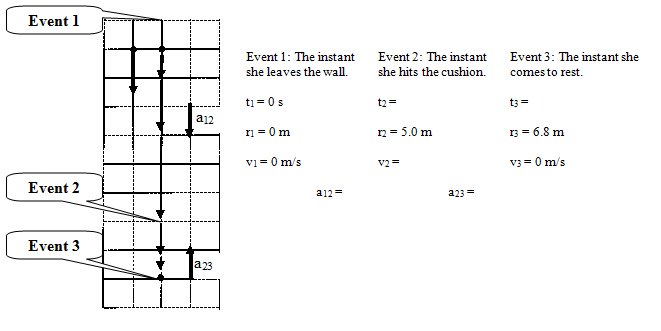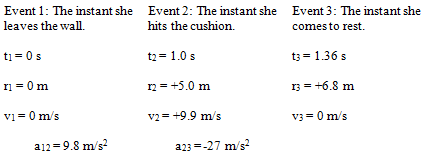# 04. Analyzing a More Complex Scenario

$$\newcommand{\vecs}{\overset { \rightharpoonup} {\mathbf{#1}} }$$ $$\newcommand{\vecd}{\overset{-\!-\!\rightharpoonup}{\vphantom{a}\smash {#1}}}$$$$\newcommand{\id}{\mathrm{id}}$$ $$\newcommand{\Span}{\mathrm{span}}$$ $$\newcommand{\kernel}{\mathrm{null}\,}$$ $$\newcommand{\range}{\mathrm{range}\,}$$ $$\newcommand{\RealPart}{\mathrm{Re}}$$ $$\newcommand{\ImaginaryPart}{\mathrm{Im}}$$ $$\newcommand{\Argument}{\mathrm{Arg}}$$ $$\newcommand{\norm}{\| #1 \|}$$ $$\newcommand{\inner}{\langle #1, #2 \rangle}$$ $$\newcommand{\Span}{\mathrm{span}}$$ $$\newcommand{\id}{\mathrm{id}}$$ $$\newcommand{\Span}{\mathrm{span}}$$ $$\newcommand{\kernel}{\mathrm{null}\,}$$ $$\newcommand{\range}{\mathrm{range}\,}$$ $$\newcommand{\RealPart}{\mathrm{Re}}$$ $$\newcommand{\ImaginaryPart}{\mathrm{Im}}$$ $$\newcommand{\Argument}{\mathrm{Arg}}$$ $$\newcommand{\norm}{\| #1 \|}$$ $$\newcommand{\inner}{\langle #1, #2 \rangle}$$ $$\newcommand{\Span}{\mathrm{span}}$$$$\newcommand{\AA}{\unicode[.8,0]{x212B}}$$

# Analyzing a More Complex Scenario

Before you start analyzing dynamics scenarios on your own, let's work our way through a more complex scenario.

To practice falling, a 55 kg pole-vaulter falls from rest off of a wall 5.0 m above a foam cushion. The pole-vaulter sinks about 1.8 m into the cushion before stopping.

Before we begin analyzing the forces acting on this pole-vaulter, I think we should try to get a handle on the kinematics of the situation. Therefore, our first step in analyzing this situation is to draw a motion diagram and tabulate motion information.Notice that between the instant she leaves the wall and the instant she hits the cushion the acceleration is positive (down), while between the instant she hits the cushion and the instant she comes to rest the acceleration is negative (up). Thus, when applying the kinematic relationships and Newton's Second Law we will have to be careful not to confuse variables between these two intervals.

What should jump out at you is the fact that this kinematic scenario can not be solved! There are five unknown kinematic quantities and only four kinematic equations. Something else is needed in order to complete the kinematic description. Let's look at the forces acting on the pole-vaulter to see if we can figure out another piece of kinematic information.

Between the first two instants, the only force acting on the pole-vaulter is the force of gravity. Once she hits the cushion, however, there are two forces acting on the pole-vaulter, the force of gravity and the force of the cushion. Correct free-body diagrams for these two distinct phases of her motion are given below.The diagram on the left corresponds to the first time interval and the diagram on the right to the second time interval. For each of these free-body diagrams, I will apply Newton's Second Law:Thus, from Newton's Second Law, we know that the acceleration during the fall is 9.8 m/s2. (We still don't know what the acceleration was during the impact portion of the motion.) Substituting this value back into the motion table yields:This is now solvable, using strictly kinematics, for the four remaining unknowns. Try to do the math on your own, and compare your result to:We now have a complete kinematic description of the motion.

Returning to Newton's Second Law for the impact portion of the motion,The cushion exerts a force of about 2000 N on the pole-vaulter to stop her fall.

This page titled 04. Analyzing a More Complex Scenario is shared under a CC BY-NC-SA license and was authored, remixed, and/or curated by Paul D'Alessandris.